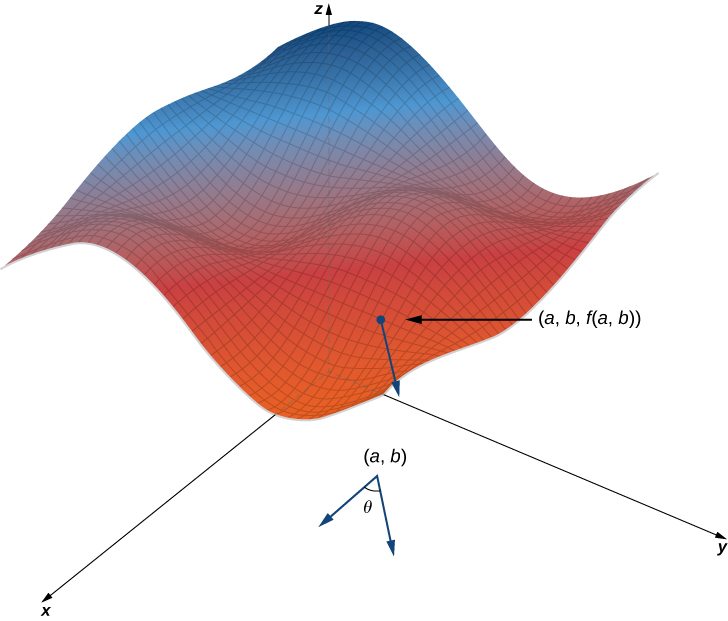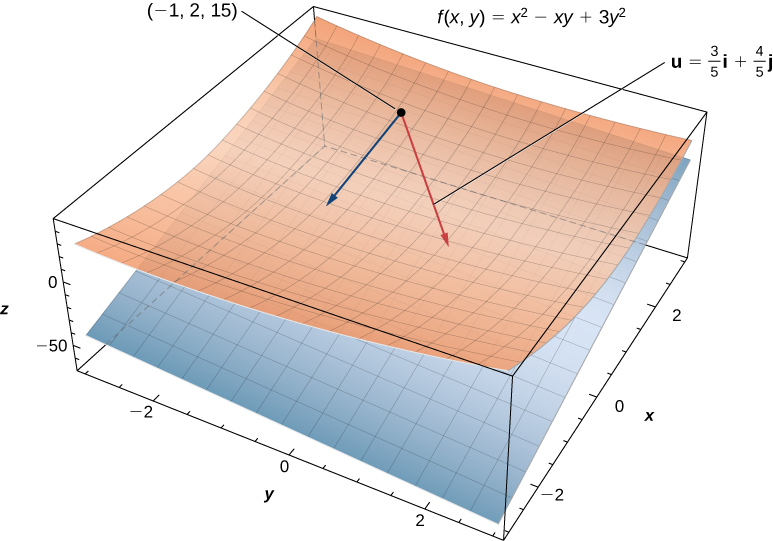4.6 Directional derivatives and the gradient

 Page 1 / 8
• Determine the directional derivative in a given direction for a function of two variables.
• Determine the gradient vector of a given real-valued function.
• Explain the significance of the gradient vector with regard to direction of change along a surface.
• Use the gradient to find the tangent to a level curve of a given function.
• Calculate directional derivatives and gradients in three dimensions.

In Partial Derivatives we introduced the partial derivative. A function $z=f\left(x,y\right)$ has two partial derivatives: $\partial z\text{/}\partial x$ and $\partial z\text{/}\partial y.$ These derivatives correspond to each of the independent variables and can be interpreted as instantaneous rates of change (that is, as slopes of a tangent line). For example, $\partial z\text{/}\partial x$ represents the slope of a tangent line passing through a given point on the surface defined by $z=f\left(x,y\right),$ assuming the tangent line is parallel to the x -axis. Similarly, $\partial z\text{/}\partial y$ represents the slope of the tangent line parallel to the $y\text{-axis.}$ Now we consider the possibility of a tangent line parallel to neither axis.

Directional derivatives

We start with the graph of a surface defined by the equation $z=f\left(x,y\right).$ Given a point $\left(a,b\right)$ in the domain of $f,$ we choose a direction to travel from that point. We measure the direction using an angle $\theta ,$ which is measured counterclockwise in the x , y -plane, starting at zero from the positive x -axis ( [link] ). The distance we travel is $h$ and the direction we travel is given by the unit vector $u=\left(\text{cos}\phantom{\rule{0.2em}{0ex}}\theta \right)i+\left(\text{sin}\phantom{\rule{0.2em}{0ex}}\theta \right)j.$ Therefore, the z -coordinate of the second point on the graph is given by $z=f\left(a+h\phantom{\rule{0.2em}{0ex}}\text{cos}\phantom{\rule{0.2em}{0ex}}\theta ,b+h\phantom{\rule{0.2em}{0ex}}\text{sin}\phantom{\rule{0.2em}{0ex}}\theta \right).$Finding the directional derivative at a point on the graph of z = f ( x , y ) . The slope of the black arrow on the graph indicates the value of the directional derivative at that point.

We can calculate the slope of the secant line by dividing the difference in $z\text{-values}$ by the length of the line segment connecting the two points in the domain. The length of the line segment is $h.$ Therefore, the slope of the secant line is

${m}_{\text{sec}}=\frac{f\left(a+h\phantom{\rule{0.2em}{0ex}}\text{cos}\phantom{\rule{0.2em}{0ex}}\theta ,b+h\phantom{\rule{0.2em}{0ex}}\text{sin}\phantom{\rule{0.2em}{0ex}}\theta \right)-f\left(a,b\right)}{h}.$

To find the slope of the tangent line in the same direction, we take the limit as $h$ approaches zero.

Definition

Suppose $z=f\left(x,y\right)$ is a function of two variables with a domain of $D.$ Let $\left(a,b\right)\in D$ and define $\text{u}=\text{cos}\phantom{\rule{0.2em}{0ex}}\theta i+\text{sin}\phantom{\rule{0.2em}{0ex}}\theta j.$ Then the directional derivative    of $f$ in the direction of $u$ is given by

${D}_{u}f\left(a,b\right)=\underset{h\to 0}{\text{lim}}\frac{f\left(a+h\phantom{\rule{0.2em}{0ex}}\text{cos}\phantom{\rule{0.2em}{0ex}}\theta ,b+h\phantom{\rule{0.2em}{0ex}}\text{sin}\phantom{\rule{0.2em}{0ex}}\theta \right)-f\left(a,b\right)}{h},$

provided the limit exists.

[link] provides a formal definition of the directional derivative that can be used in many cases to calculate a directional derivative.

Finding a directional derivative from the definition

Let $\theta =\text{arccos}\left(3\text{/}5\right).$ Find the directional derivative ${D}_{u}f\left(x,y\right)$ of $f\left(x,y\right)={x}^{2}-xy+3{y}^{2}$ in the direction of $\text{u}=\left(\text{cos}\phantom{\rule{0.2em}{0ex}}\theta \right)i+\left(\text{sin}\phantom{\rule{0.2em}{0ex}}\theta \right)j.$ What is ${D}_{\text{u}}f\left(-1,2\right)?$

First of all, since $\text{cos}\phantom{\rule{0.2em}{0ex}}\theta =3\text{/}5$ and $\theta$ is acute, this implies

$\text{sin}\phantom{\rule{0.2em}{0ex}}\theta =\sqrt{1-{\left(\frac{3}{5}\right)}^{2}}=\sqrt{\frac{16}{25}}=\frac{4}{5}.$

Using $f\left(x,y\right)={x}^{2}-xy+3{y}^{2},$ we first calculate $f\left(x+h\phantom{\rule{0.2em}{0ex}}\text{cos}\phantom{\rule{0.2em}{0ex}}\theta ,y+h\phantom{\rule{0.2em}{0ex}}\text{sin}\phantom{\rule{0.2em}{0ex}}\theta \right)\text{:}$

$\begin{array}{cc}\hfill f\left(x+h\phantom{\rule{0.2em}{0ex}}\text{cos}\phantom{\rule{0.2em}{0ex}}\theta ,y+h\phantom{\rule{0.2em}{0ex}}\text{sin}\phantom{\rule{0.2em}{0ex}}\theta \right)& ={\left(x+h\phantom{\rule{0.2em}{0ex}}\text{cos}\phantom{\rule{0.2em}{0ex}}\theta \right)}^{2}-\left(x+h\phantom{\rule{0.2em}{0ex}}\text{cos}\phantom{\rule{0.2em}{0ex}}\theta \right)\left(y+h\phantom{\rule{0.2em}{0ex}}\text{sin}\phantom{\rule{0.2em}{0ex}}\theta \right)+3{\left(y+h\phantom{\rule{0.2em}{0ex}}\text{sin}\phantom{\rule{0.2em}{0ex}}\theta \right)}^{2}\hfill \\ & ={x}^{2}+2xh\phantom{\rule{0.2em}{0ex}}\text{cos}\phantom{\rule{0.2em}{0ex}}\theta +{h}^{2}{\text{cos}}^{2}\theta -xy-xh\phantom{\rule{0.2em}{0ex}}\text{sin}\phantom{\rule{0.2em}{0ex}}\theta -yh\phantom{\rule{0.2em}{0ex}}\text{cos}\phantom{\rule{0.2em}{0ex}}\theta \hfill \\ & \phantom{\rule{0.5em}{0ex}}{\mathit{\text{−h}}}^{2}\text{sin}\phantom{\rule{0.2em}{0ex}}\theta \phantom{\rule{0.2em}{0ex}}\text{cos}\phantom{\rule{0.2em}{0ex}}\theta +3{y}^{2}+6yh\phantom{\rule{0.2em}{0ex}}\text{sin}\phantom{\rule{0.2em}{0ex}}\theta +3{h}^{2}{\text{sin}}^{2}\theta \hfill \\ & ={x}^{2}+2xh\left(\frac{3}{5}\right)+\frac{9{h}^{2}}{25}-xy-\frac{4xh}{5}-\frac{3yh}{5}-\frac{12{h}^{2}}{25}+3{y}^{2}\hfill \\ & \phantom{\rule{0.5em}{0ex}}+6yh\left(\frac{4}{5}\right)+3{h}^{2}\left(\frac{16}{25}\right)\hfill \\ & ={x}^{2}-xy+3{y}^{2}+\frac{2xh}{5}+\frac{9{h}^{2}}{5}+\frac{21yh}{5}.\hfill \end{array}$

We substitute this expression into [link] :

$\begin{array}{cc}\hfill {D}_{u}f\left(a,b\right)& =\underset{h\to 0}{\text{lim}}\frac{f\left(a+h\phantom{\rule{0.2em}{0ex}}\text{cos}\phantom{\rule{0.2em}{0ex}}\theta ,b+h\phantom{\rule{0.2em}{0ex}}\text{sin}\phantom{\rule{0.2em}{0ex}}\theta \right)-f\left(a,b\right)}{h}\hfill \\ & =\underset{h\to 0}{\text{lim}}\frac{\left({x}^{2}-xy+3{y}^{2}+\frac{2xh}{5}+\frac{9{h}^{2}}{5}+\frac{21yh}{5}\right)-\left({x}^{2}-xy+3{y}^{2}\right)}{h}\hfill \\ & =\underset{h\to 0}{\text{lim}}\frac{\frac{2xh}{5}+\frac{9{h}^{2}}{5}+\frac{21yh}{5}}{h}\hfill \\ & =\underset{h\to 0}{\text{lim}}\frac{2x}{5}+\frac{9h}{5}+\frac{21y}{5}\hfill \\ & =\frac{2x+21y}{5}.\hfill \end{array}$

To calculate ${D}_{u}f\left(-1,2\right),$ we substitute $x=-1$ and $y=2$ into this answer:

$\begin{array}{cc}\hfill {D}_{\text{u}}f\left(-1,2\right)& =\frac{2\left(-1\right)+21\left(2\right)}{5}\hfill \\ & =\frac{-2+42}{5}\hfill \\ & =8.\hfill \end{array}$

(See the following figure.)Finding the directional derivative in a given direction u at a given point on a surface. The plane is tangent to the surface at the given point ( −1 , 2 , 15 ) .

Is there any normative that regulates the use of silver nanoparticles?
what king of growth are you checking .?
Renato
What fields keep nano created devices from performing or assimulating ? Magnetic fields ? Are do they assimilate ?
why we need to study biomolecules, molecular biology in nanotechnology?
?
Kyle
yes I'm doing my masters in nanotechnology, we are being studying all these domains as well..
why?
what school?
Kyle
biomolecules are e building blocks of every organics and inorganic materials.
Joe
anyone know any internet site where one can find nanotechnology papers?
research.net
kanaga
sciencedirect big data base
Ernesto
Introduction about quantum dots in nanotechnology
what does nano mean?
nano basically means 10^(-9). nanometer is a unit to measure length.
Bharti
do you think it's worthwhile in the long term to study the effects and possibilities of nanotechnology on viral treatment?
absolutely yes
Daniel
how to know photocatalytic properties of tio2 nanoparticles...what to do now
it is a goid question and i want to know the answer as well
Maciej
Abigail
for teaching engĺish at school how nano technology help us
Anassong
Do somebody tell me a best nano engineering book for beginners?
there is no specific books for beginners but there is book called principle of nanotechnology
NANO
what is fullerene does it is used to make bukky balls
are you nano engineer ?
s.
fullerene is a bucky ball aka Carbon 60 molecule. It was name by the architect Fuller. He design the geodesic dome. it resembles a soccer ball.
Tarell
what is the actual application of fullerenes nowadays?
Damian
That is a great question Damian. best way to answer that question is to Google it. there are hundreds of applications for buck minister fullerenes, from medical to aerospace. you can also find plenty of research papers that will give you great detail on the potential applications of fullerenes.
Tarell
what is the Synthesis, properties,and applications of carbon nano chemistry
Mostly, they use nano carbon for electronics and for materials to be strengthened.
Virgil
is Bucky paper clear?
CYNTHIA
carbon nanotubes has various application in fuel cells membrane, current research on cancer drug,and in electronics MEMS and NEMS etc
NANO
so some one know about replacing silicon atom with phosphorous in semiconductors device?
Yeah, it is a pain to say the least. You basically have to heat the substarte up to around 1000 degrees celcius then pass phosphene gas over top of it, which is explosive and toxic by the way, under very low pressure.
Harper
Do you know which machine is used to that process?
s.
how to fabricate graphene ink ?
for screen printed electrodes ?
SUYASH
What is lattice structure?
of graphene you mean?
Ebrahim
or in general
Ebrahim
in general
s.
Graphene has a hexagonal structure
tahir
On having this app for quite a bit time, Haven't realised there's a chat room in it.
Cied
what is biological synthesis of nanoparticles
how did you get the value of 2000N.What calculations are needed to arrive at it
Privacy Information Security Software Version 1.1a
Good
Berger describes sociologists as concerned with
Got questions? Join the online conversation and get instant answers!By Anonymous UserBy Abishek DevarajBy Sebastian Sieczko...ByBy